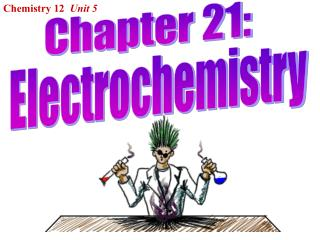DownloadDownload PresentationChemistry 12 Unit 5

Chemistry 12 Unit 5

Télécharger la présentationChemistry 12 Unit 5

- - - - - - - - - - - - - - - - - - - - - - - - - - - E N D - - - - - - - - - - - - - - - - - - - - - - - - - - -
Presentation Transcript

1. Chemistry 12 Unit 5 Chapter 21: Electrochemistry

2. Unit V: Electrochemistry Oxidation – Reduction Reactions: Oxidation: A substance losing electrons. Reduction: A substance gaining electrons. eg: Al(s) in CuCl2(aq) Al(s) + CuCl2(aq)  Cu(s) + AlCl3(aq) (not balanced) Net Ionic Equation: Al(s) + Cu2+(aq)  Cu(s) + Al3+(aq) Reduction Half-Reaction: Cu2+(aq) + 2e- Cu(s) • The reaction shows that Cu2+ wants electrons more than Al3+ Oxidation Half-Reaction: Al(s) Al3+(aq) + 3e-

3. This type of reaction is called an “oxidation – reduction” reaction or a “redox” reaction. • Aluminum metal is oxidized and copper (II) ions are reduced. • The complete redox equation consists of some multiple of the two half-reactions: 3 ( ) Cu2+(aq) + 2e- Cu(s) 2 ( ) Al(s) Al3+(aq) + 3e- • This shows the same number of electrons gained as lost: 2Al(s) + 3Cu2+(aq)+ 6e- 2Al3+(aq) + 3Cu(s)+ 6e- Balanced Redox Equation: 2Al(s) + 3Cu2+(aq)  2Al3+(aq) + 3Cu(s)

4. Always check that the total charge on each side of the equation is equal. 2Al(s) + 3Cu2+(aq)  2Al3+(aq) + 3Cu(s) 3(2+) = 2(3+) Terminology: In this example: Cu2+ is the oxidizing agent. Al is the reducing agent. Cu2+ is reduced to Cu. Al is oxidized to Al3+.

5. Redox Practice I: For each of the following, which substance is oxidized and which substance is reduced? Substance Oxidized Substance Reduced • Zn + CuSO4  ZnSO4 + Cu • CrCl3 + Sn Cr + SnCl4 • Fe + CuSO4  FeSO4 + Cu • MgCl2 + Cr  Mg + CrCl3 • Al + Pb(NO3)2 Al(NO3)3 + Pb

6. Redox Practice II: For each of the following, which substance is the oxidizing agent and which is the reducing agent? Oxidizing Agent Reducing Agent • CuSO4 + Zn  ZnSO4 + Cu • Sn + CrCl3 Cr + SnCl4 • Fe + CuSO4  FeSO4 + Cu • Mg + CrCl3  Cr + MgCl2 • Al(NO3)3 + Pb  Pb(NO3)2+ Al